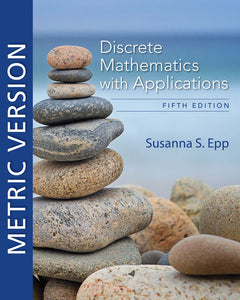# INTL DISCRETE MATHEMATICS W/APPLICATIONS METRIC VERSION

Epp

Format: Print Book

ISBN: 9780357114087

• \$57.84
Unit price per
• Save \$3.05
Tax included.

Known for its accessible, precise approach, Epp's DISCRETE MATHEMATICS WITH APPLICATIONS, 5th Edition, Metric Edition introduces discrete mathematics with clarity and precision. Coverage emphasizes the major themes of discrete mathematics as well as the reasoning that underlies mathematical thought. Students learn to think abstractly as they study the ideas of logic and proof. While learning about logic circuits and computer addition, algorithm analysis, recursive thinking, computability, automata, cryptography and combinatorics, students discover that ideas of discrete mathematics underlie and are essential to todayâ€™s science and technology. The authorâ€™s emphasis on reasoning provides a foundation for computer science and upper-level mathematics courses.

Edition5E
LanguageEnglish
Number of Pages984